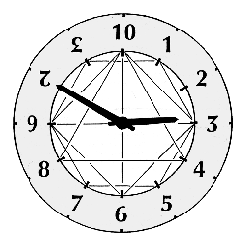# Time Units

The basic unit of time is the Mean Solar Day (MSD)

There are two main schemes for the creation of time units using base twelve.

• Dividing the MSD, as now, into two dozen hours (retaining the present hour unit)
• Dividing the MSD into twelve "double hours" (also called "duhours", "biheures")

Taking the first of these schemes (which is a part of the TGM system) we have the following units:

Hour*Timminutesseconds*seconds
1*10000603600*2100
*0·1*10005300*210
*0·01*1000·416..25*21
*0·001*100·0347..2·083..*2·1
*0·0001*10·00289..0·17361..*0·21

As now, with the "twenty-four hour clock" we can express time with two dozenal places - e.g. "a quarter past three (am)" will be *03·30 (present 03:15) and "a quarter past three (pm)" will be *13·30 (present 15:15).

Apart from the Tim and the Hour no names have been given to the other units, though we received one suggestion some time ago - "tekon" for *10Tm. "Minute" and "second" will hardly do (*0·01Hr is nearer to half a minute). Something to think about?

For the second scheme no names have yet been suggested, apart from "duor" for the double-hour, and the division of the day goes:

Dayseconds (dec)seconds (doz)
12^-60·02893518330·042
12^-50·3472222 0·42
12^-44·1666666 4·2
12^-35042
12^-2600420
12^-172004200
12^08640042000

### by uaxtecum

It seems clear to me that dividing the circle into twelve parts makes more sense than dividing half a circle.

Not only a full circle seems the natural unit to be subdivided according to the base of numeration, with other choices like half a circle, a quarter of a circle (like the French revolutionaries), or a sixth of a circle (like the Babylonians), seeming rather arbitrary, even though 180, 90 and 60 are, of course, very important angles in geometry. To start with, one speaks of a "full circle", "half a circle", a "quarter of a circle", etc., and not of a "double halfcircle", a "full halfcircle", "half a halfcircle" or the like, which means it's the full circle and not half a circle what's being conceptualized as a unit.

Also, the fundamental trigonometric functions sine and cosine have a period equal to a full circle, not half a circle (that's the period of the less elementary tangent and cotangent). It is thus a disadvantage that the definition of radian makes a full circle have 2π radians instead of π radians (a consequence of the inconsistency of having π defined in terms of diameter, but the radian in terms of radius), so that you need to halve the number of radians in order to get how many turns or sine/cosine periods it represents. I think this makes things more confusing than they could be.

Moreover, have you considered that one twelfth of a circle is precisely the very important angle of 30 sexagesimal degrees? That's the angle at which sine equals one half; also the one you get by bisecting the angles of an equilateral triangle (which means drawing its altitudes, which intersect at its centroid), apart from the angle between two adjacent hours in a clock face. One twelfth of half a circle (or of a quarter, or of a sixth) has no similar geometrical or mathematical significance.

Finally, one radian is *0·1TE02T1443579... times a full circle, in dozenal notation. That is, you can get a fairly good approximation (with an error under *0·016%) by rounding it to *1TE degrees if you make sin *100 = 1/2, sin *160 = cos *160, cos *200 = 1/2, *300 a right angle, *600 a flat angle, and *1,000 a full turn. If the radian were redefined as the angle subtended by an arc of length equal to the diameter instead of the radius (and so, maybe renamed to diametrian), then it would equal *0·39T0582886E36... times a full circle; that is, easily rounded to *39A of said dozenal degrees (same pergross error).

Following this, if the day is divided into *10 double-hours (as done by the Sumerians) of *100 quasi-minutes (1 of these = fifty seconds), then each double-hour would represent a turn of *100 degrees (thirty degrees sexagesimal) and one quasi-minute a *1 degree turn of the Earth around its axis. I think this is a very convenient way to harmonize angle and time measures, and one that is consistently dozenal.